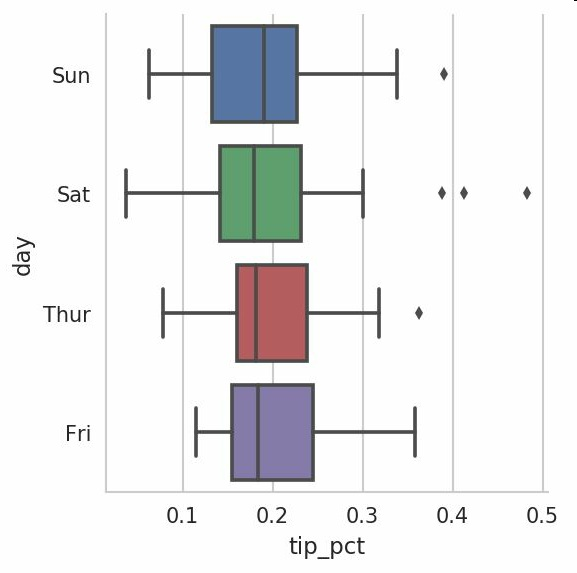# 绘图和可视化

matplotlib是用于创建出版质量图标的桌面绘图包（主要是2D方面）。matplotlib和IPython社区进行合作，简化了从IPython shell进行交互式绘图。matplotlib支持各种操作系统上许多不同的GUI后端，而且还能将图片导出为各种常见的矢量和光栅图：PDF、SVG、JPG、PNG、BMP和GIF等。

``````%matplotlib notebook
``````
• 1

## 1.matplotlib入门

matplotlib的通常引入约定是：

``````In : import matplotlib.pyplot as plt
``````
• 1

``````In : import numpy as np
In : data = np.arange(10)
In : data
Out: array([0, 1, 2, 3, 4, 5, 6, 7, 8, 9])
In : plt.plot(data)
``````
• 1
• 2
• 3
• 4
• 5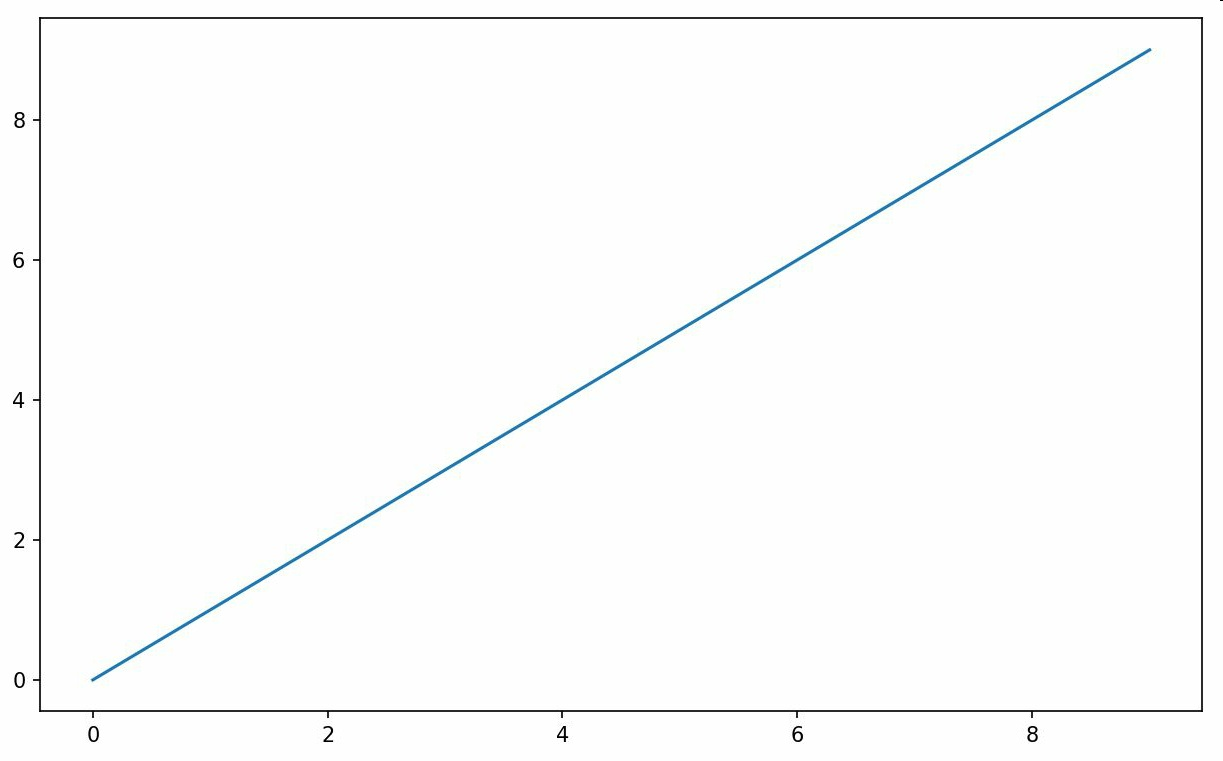### Figure和Subplot

matplotlib的图像位于Figure中，可以使用plt.figure创建一个新的Figure：

``````In : fig = plt.figure()
``````
• 1

``````In : ax1 = fig.add_subplot(2, 2, 1)
In : ax2 = fig.add_subplot(2, 2, 2)
In : ax3 = fig.add_subplot(2, 2, 3)
``````
• 1
• 2
• 3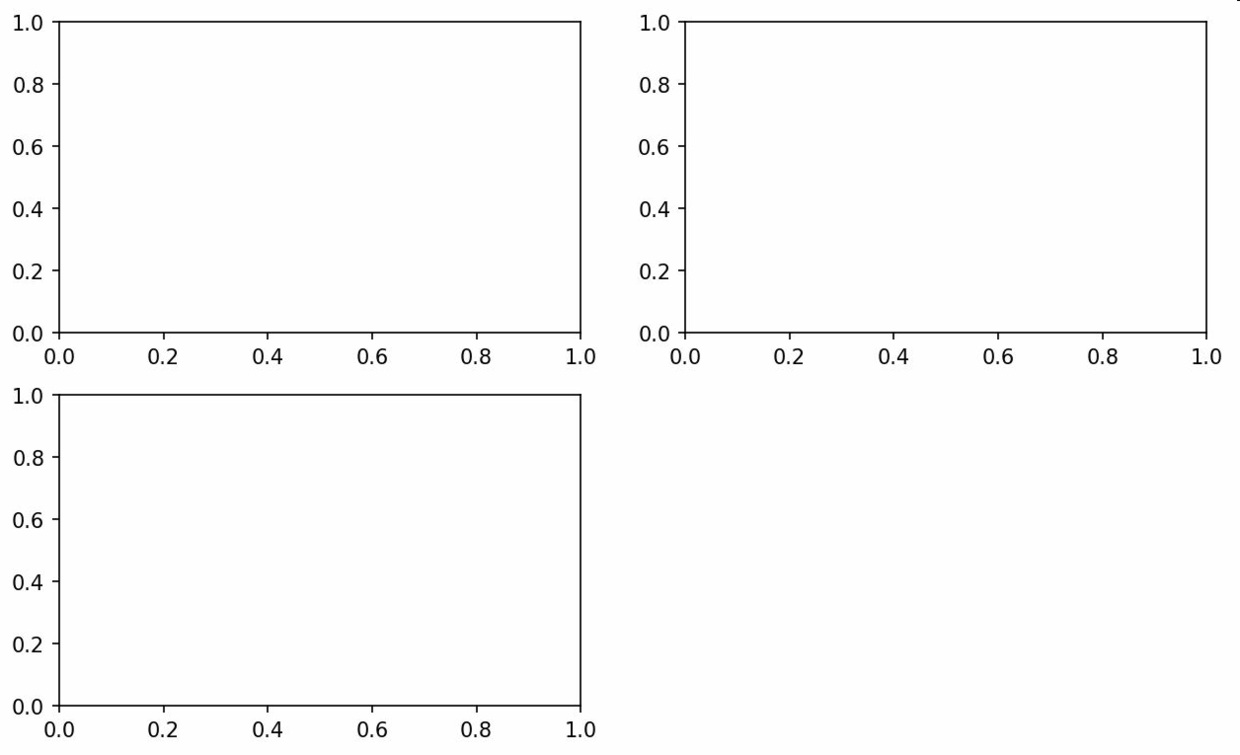``````In : plt.plot(np.random.randn(50).cumsum(), 'k--')
In : ax1.hist(np.random.randn(100), bins=20, color='k', alph
a=0.3)
In : ax2.scatter(np.arange(30), np.arange(30) + 3 * np.rando
m.randn(30))
``````
• 1
• 2
• 3
• 4
• 5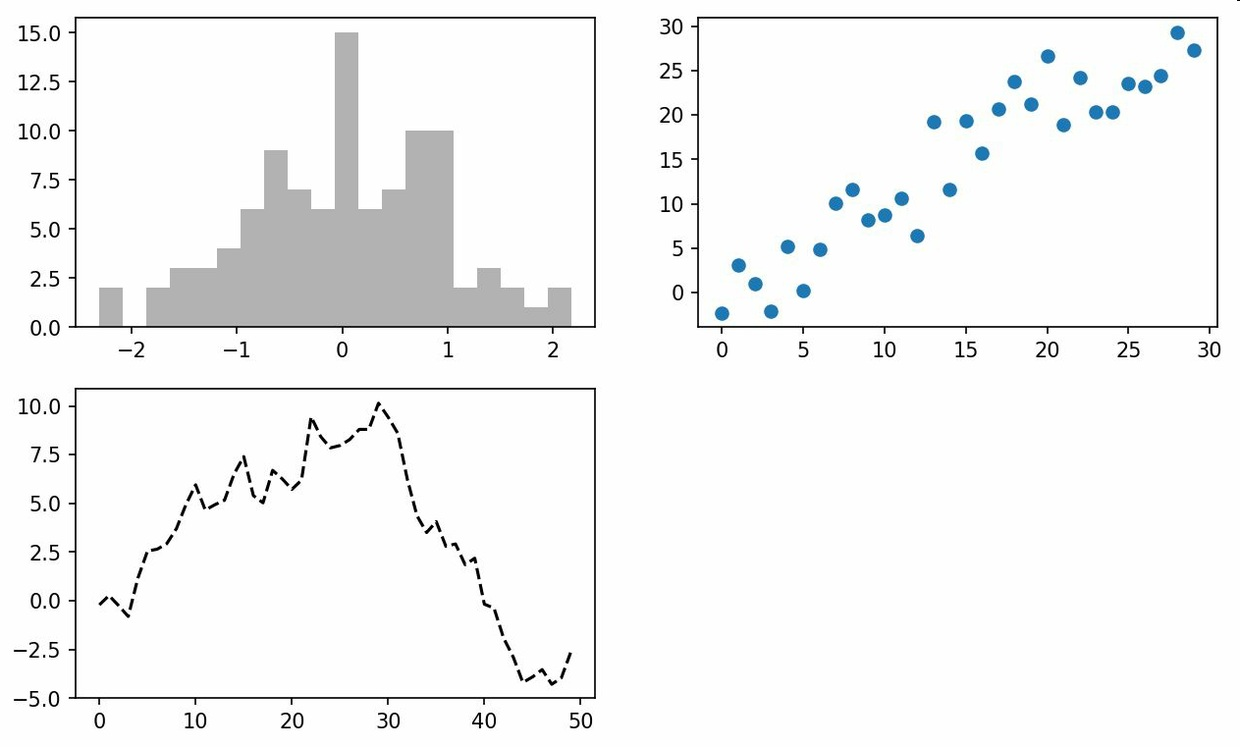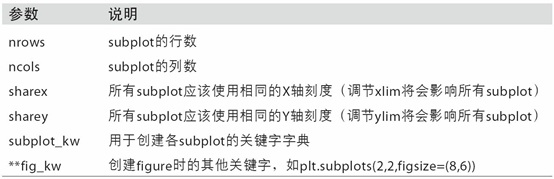### 调整subplot周围的间距

``````subplots_adjust(left=None, bottom=None, right=None, top=None,
wspace=None, hspace=None)
``````
• 1
• 2

wspace和hspace用于控制宽度和高度的百分比，可以用做subplot之间的额间距

``````fig, axes = plt.subplots(2, 2, sharex=True, sharey=True)
for i in range(2):
for j in range(2):
axes[i, j].hist(np.random.randn(500), bins=50, color='k'
, alpha=0.5)
plt.subplots
_
``````
• 1
• 2
• 3
• 4
• 5
• 6
• 7
• 8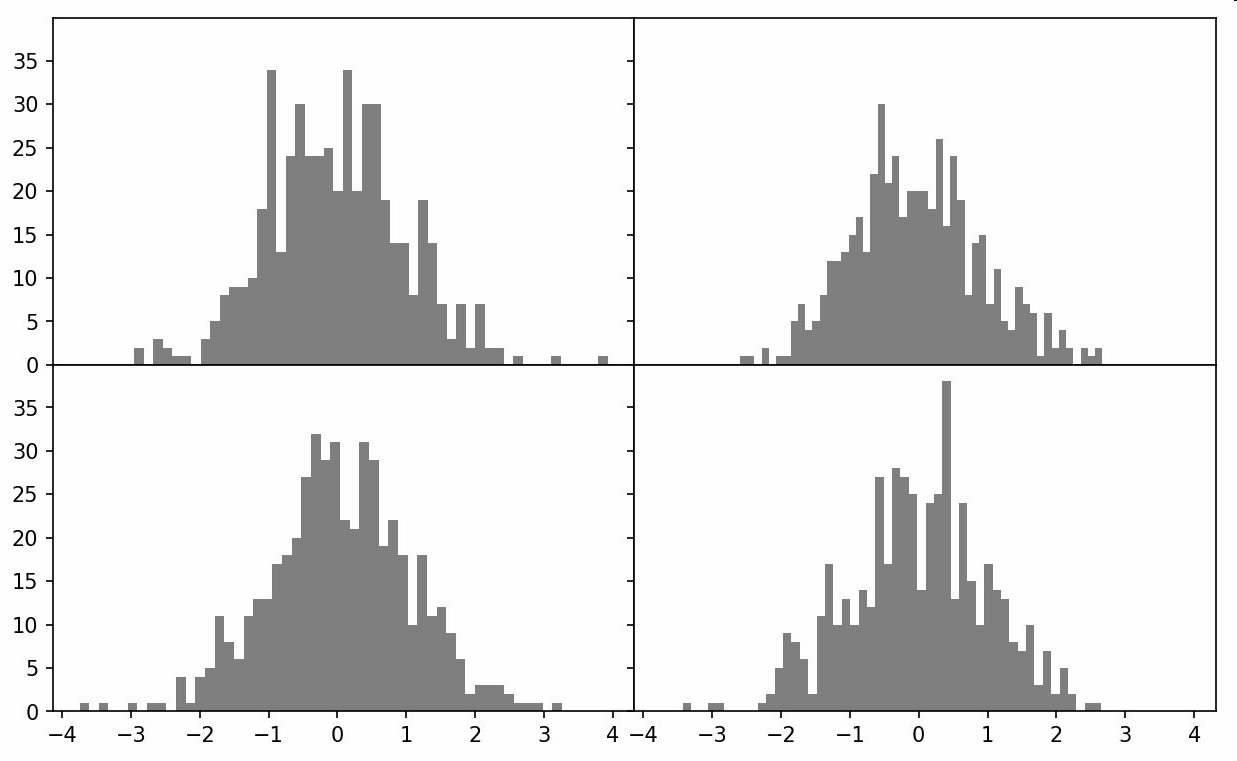### 颜色、标记和线型

matplotlib的plot函数接受一组X和Y坐标，还可以接受一个表示颜色和线型的字符串缩写，如要分局X和Y绘制徐色虚线，则：

``````ax.plot(x, y, 'g--')
``````
• 1

``````ax.plot(x, y, linestyle='--', color='g')
``````
• 1

### 刻度、标签和图例

pyplot接口的设计目的是交互式使用，含有注入xlim、xticks和xticklabels之类的方法，分别控制图表的范围、刻度位置、刻度标签等。其使用方式有一些两种：
1）调用时不带参数，则返回当前的参数值；
2）调用时带参数，则设置参数值

### 设置标题、轴标签、刻度以及刻度标签

``````In : fig = plt.figure()
In : ax = fig.add_subplot(1, 1, 1)
In : ax.plot(np.random.randn(1000).cumsum())
``````
• 1
• 2
• 3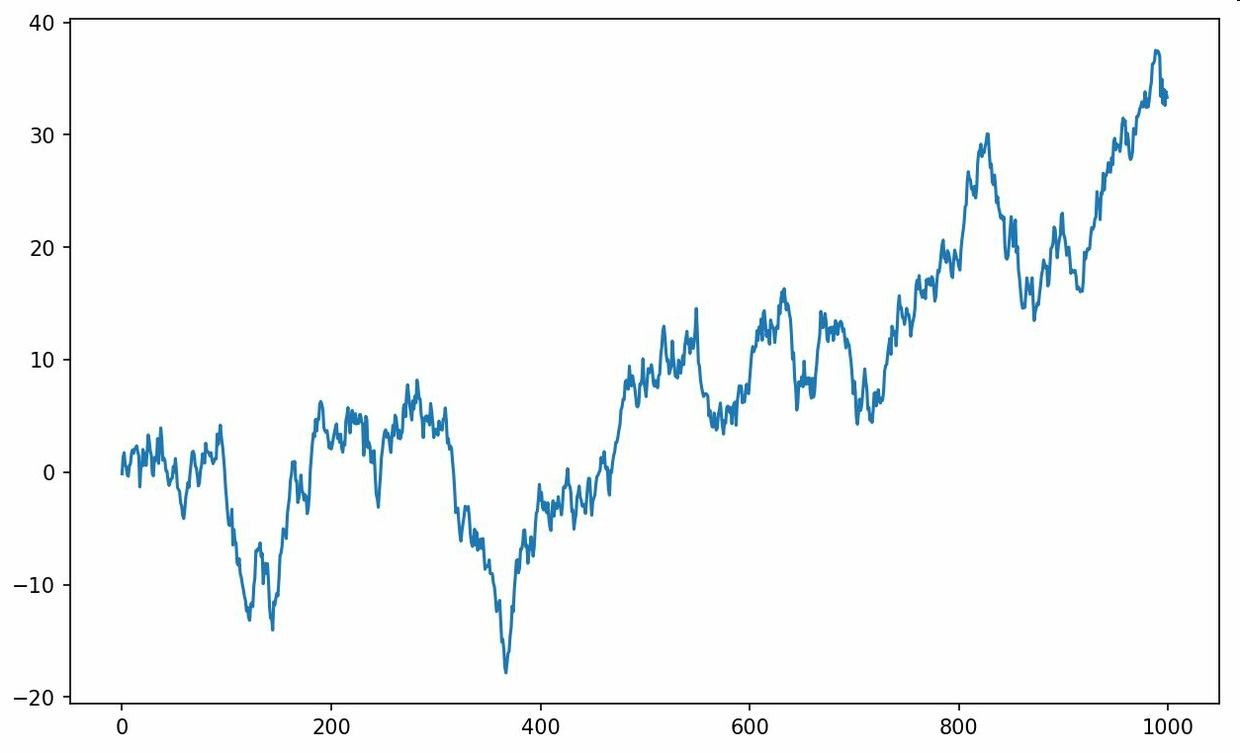``````In : ticks = ax.set_xticks([0, 250, 500, 750, 1000])
In : labels = ax.set_xticklabels(['one', 'two', 'three', 'four', 'five'],
....: rotation=30, fontsize='small')
``````
• 1
• 2
• 3

rotation选项设定X刻度标签倾斜30度，再用set_xlabel为X轴设置一个名称，并用set_title设置一个标题：

``````In : ax.set
_
title('My first matplotlib plot')
Out: <matplotlib.text.Text at 0x7fb624d055f8>
In : ax.set
_
xlabel('Stages')
``````
• 1
• 2
• 3
• 4
• 5
• 6
• 7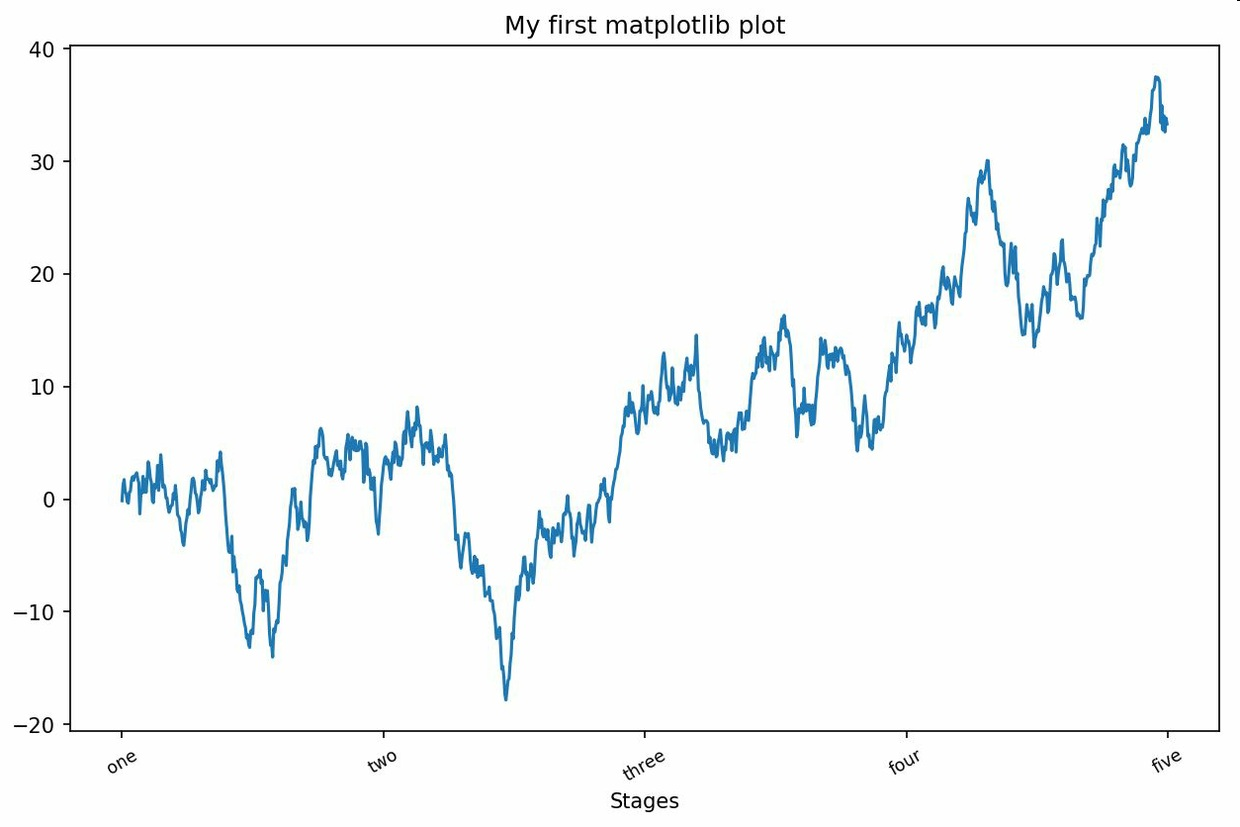### 添加图例

``````In : from numpy.random import randn
In : fig = plt.figure(); ax = fig.add
_
subplot(1, 1, 1)
In : ax.plot(randn(1000).cumsum(), 'k', label='one')
Out: [<matplotlib.lines.Line2D at 0x7fb624bdf860>]
In : ax.plot(randn(1000).cumsum(), 'k--', label='two')
Out: [<matplotlib.lines.Line2D at 0x7fb624be90f0>]
In : ax.plot(randn(1000).cumsum(), 'k.', label='three')
Out: [<matplotlib.lines.Line2D at 0x7fb624be9160>]
``````
• 1
• 2
• 3
• 4
• 5
• 6
• 7
• 8
• 9
• 10

``````In : ax.legend(loc='best')
``````
• 1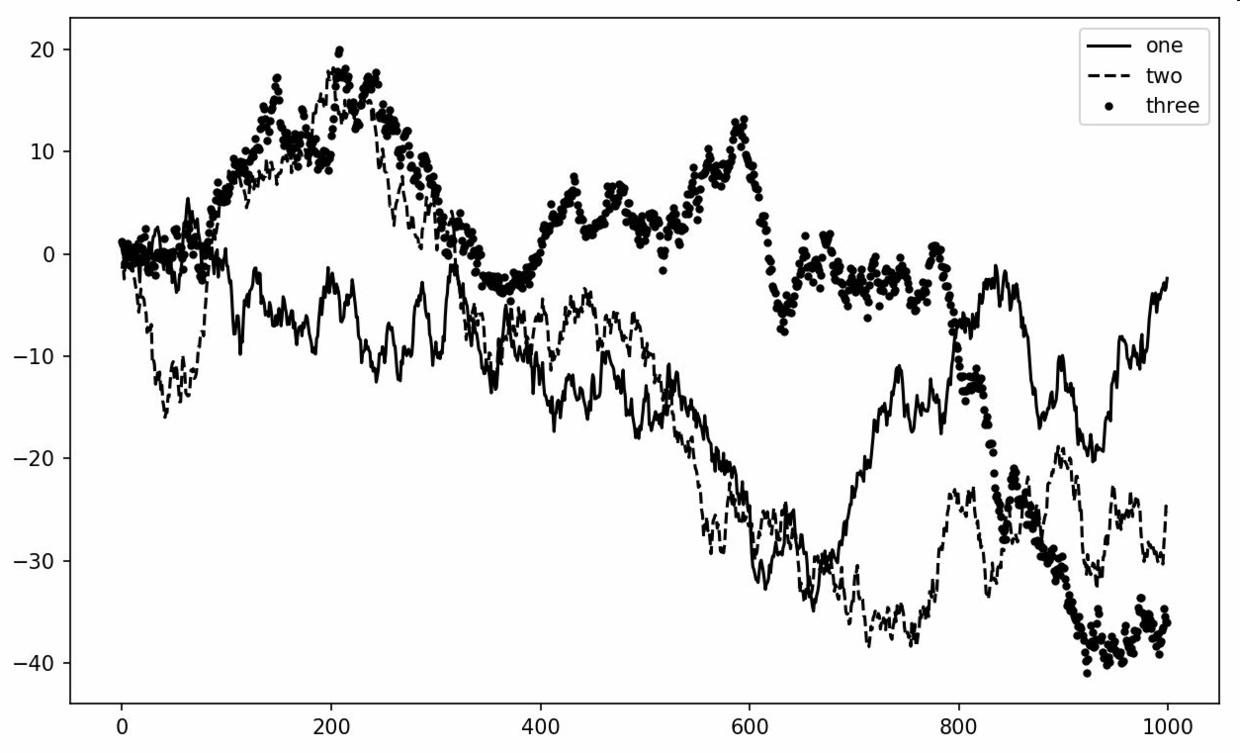### 注解以及在Subplot上绘图

``````ax.text(x, y, 'Hello world!',
family='monospace', fontsize=10)
``````
• 1
• 2

### 将图标保存到文件

``````plt.savefig('figpath.svg')
``````
• 1

``````plt.savefig('figpath.png', dpi=400, bbox_inches='tight')
``````
• 1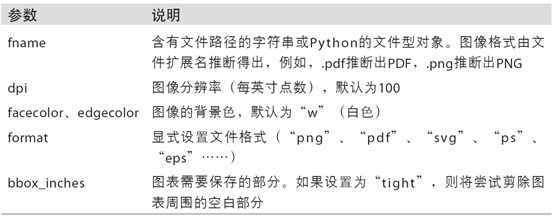### matplotlib配置

matplotlib自带一些配色方案，以及为生成出版质量的图片而设定的默认配置信息，包括管理图像大小、subplot边距、字体大小、网格类型等。一般可以使用系统的方法rc

``````plt.rc('figure', figsize=(10, 10))
``````
• 1

rc的第一个参数是自定义的对象，如’figure’,‘axex’,‘xtick’,‘grid’,’legend’等。气候可以跟上一系列的关键字参数。一个简单的方法是将这些选项写成一个字典：

``````font
_
options = {'family' : 'monospace',
'weight' : 'bold',
'size' : 'small'}
plt.rc('font',
**font
_
options)
``````
• 1
• 2
• 3
• 4
• 5
• 6
• 7
• 8
• 9

## 2.使用pandas和seaborn绘图

maplotlib是一种比较低级的工具，要绘制一张图表，包括多列数据，还有行和列标签。因此引入seaborn。

### 线形图

Series和DataFrame都有一个用于生成各类图表的plot方法，默认情况下，它们生成的是线形图

``````In : s = pd.Series(np.random.randn(10).cumsum(), index=np.ar
ange(0, 100, 10))
In : s.plot()
``````
• 1
• 2
• 3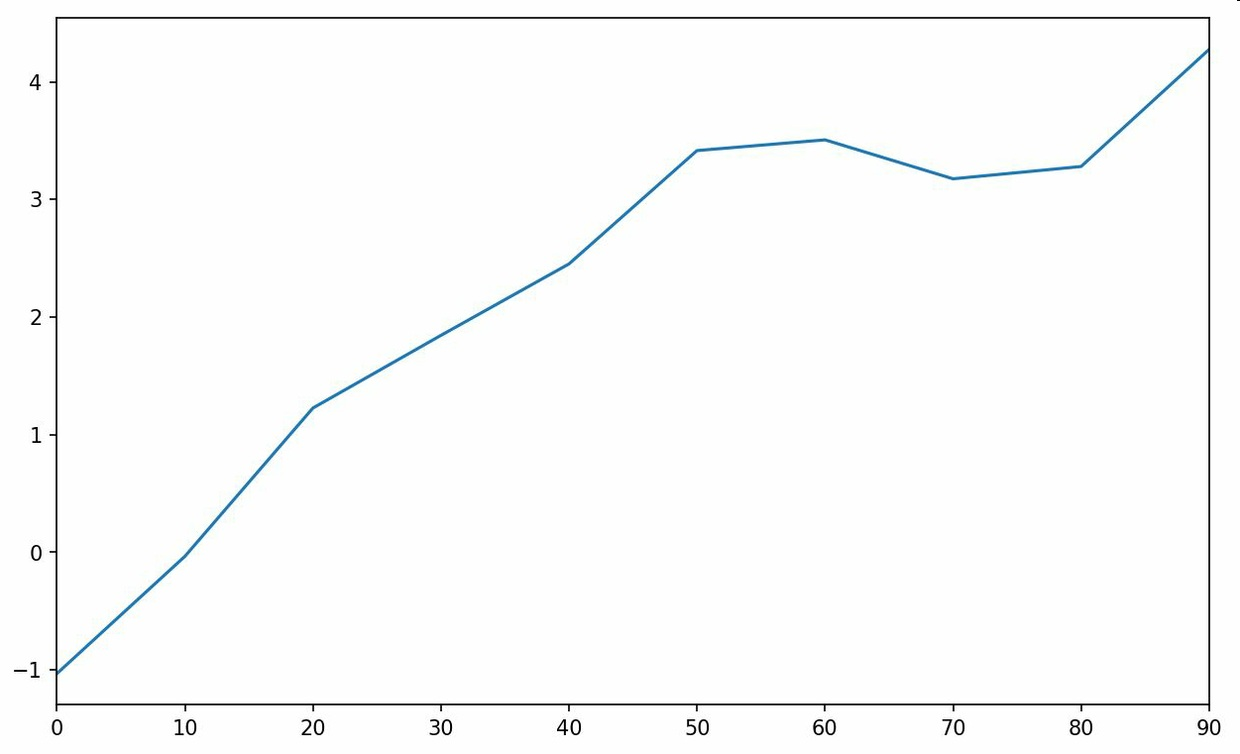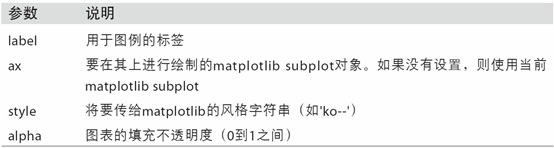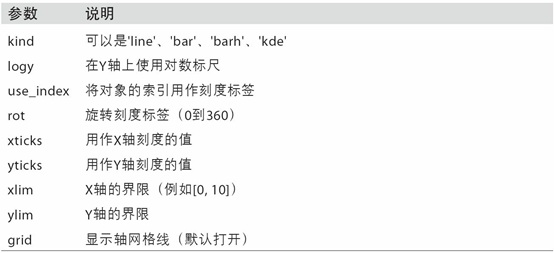pandas的大部分绘图方法都有一个可选的ax参数，是一个matplotlib的subplot对象。DataFrame的plot方法会在一个subplot中为各列绘制一条线：

``````In : df = pd.DataFrame(np.random.randn(10, 4).cumsum(0),
....: columns=['A', 'B', 'C', 'D'],
....: index=np.arange(0, 100, 10))
In : df.plot()
``````
• 1
• 2
• 3
• 4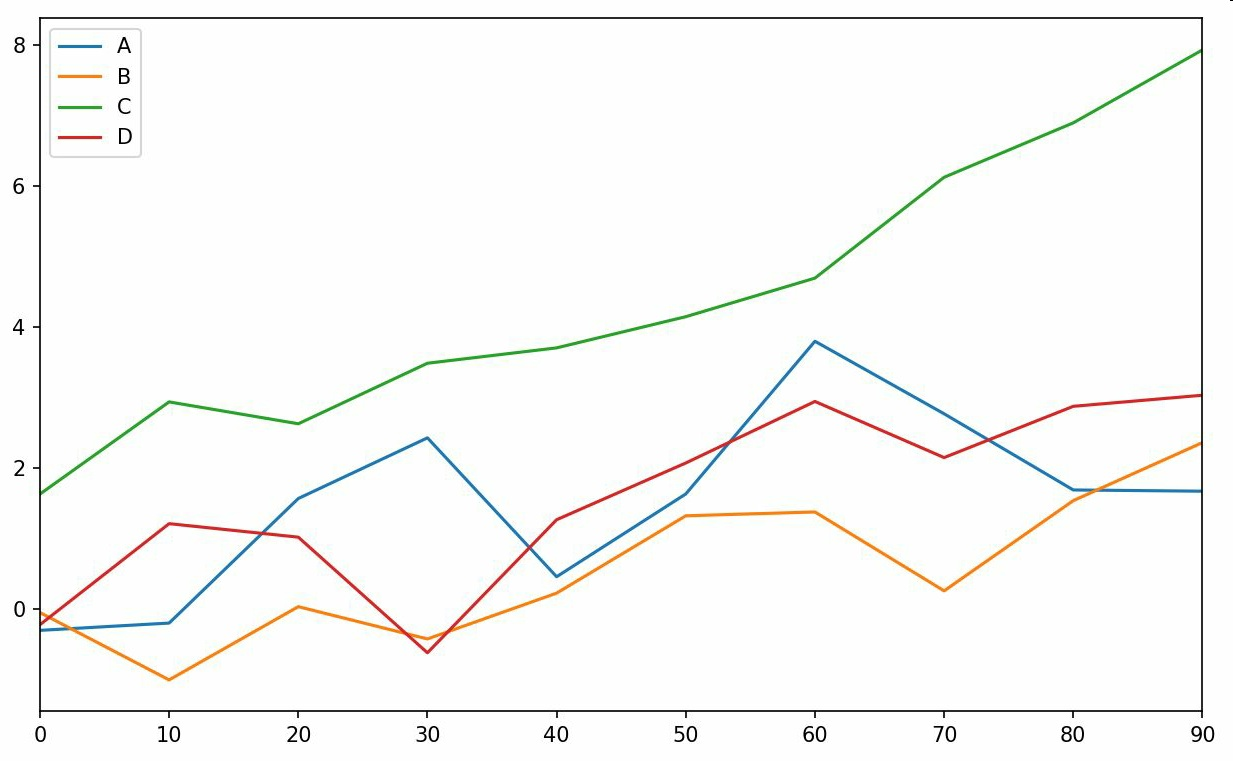DataFrame还有一些用于对列进行零号处理的选项，详细信息参照系下表：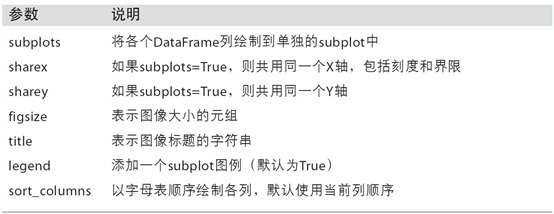### 柱状图

plot.bar()和plot.barh()分别绘制水平和垂直的柱状图。

``````In : fig, axes = plt.subplots(2, 1)
In : data = pd.Series(np.random.rand(16), index=list('abcdef
ghijklmnop'))
In : data.plot.bar(ax=axes, color='k', alpha=0.7)
Out: <matplotlib.axes._
subplots.AxesSubplot at 0x7fb62493d470
>
In : data.plot.barh(ax=axes, color='k', alpha=0.7)
``````
• 1
• 2
• 3
• 4
• 5
• 6
• 7
• 8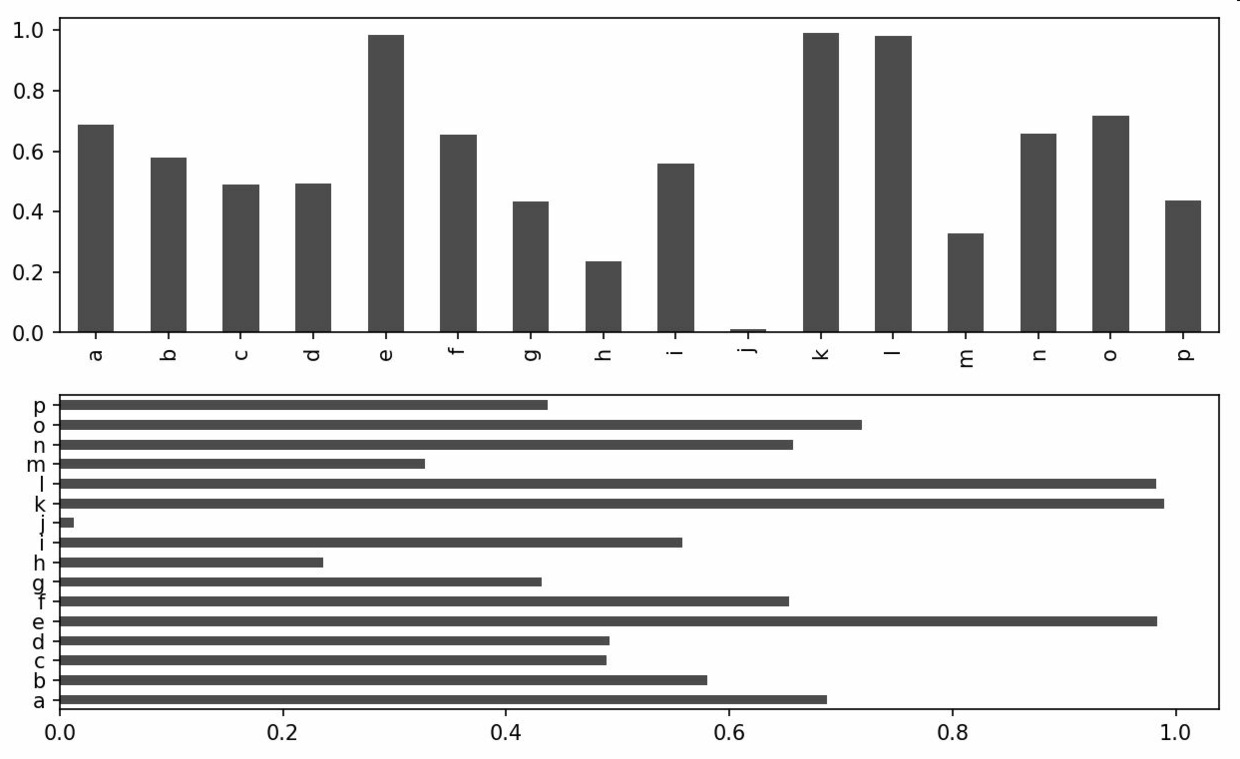color=’k’和alpha=0.7设定了图形的颜色为黑色，并使用部分的填充透明度

``````In : df = pd.DataFrame(np.random.rand(6, 4),
....: index=['one', 'two', 'three', 'four',
'five', 'six'],
....: columns=pd.Index(['A', 'B', 'C', 'D']
, name='Genus'))
In : df
Out:
Genus A B C D
one 0.370670 0.602792 0.229159 0.486744
two 0.420082 0.571653 0.049024 0.880592
three 0.814568 0.277160 0.880316 0.431326
four 0.374020 0.899420 0.460304 0.100843
five 0.433270 0.125107 0.494675 0.961825
six 0.601648 0.478576 0.205690 0.560547
In : df.plot.bar()
``````
• 1
• 2
• 3
• 4
• 5
• 6
• 7
• 8
• 9
• 10
• 11
• 12
• 13
• 14
• 15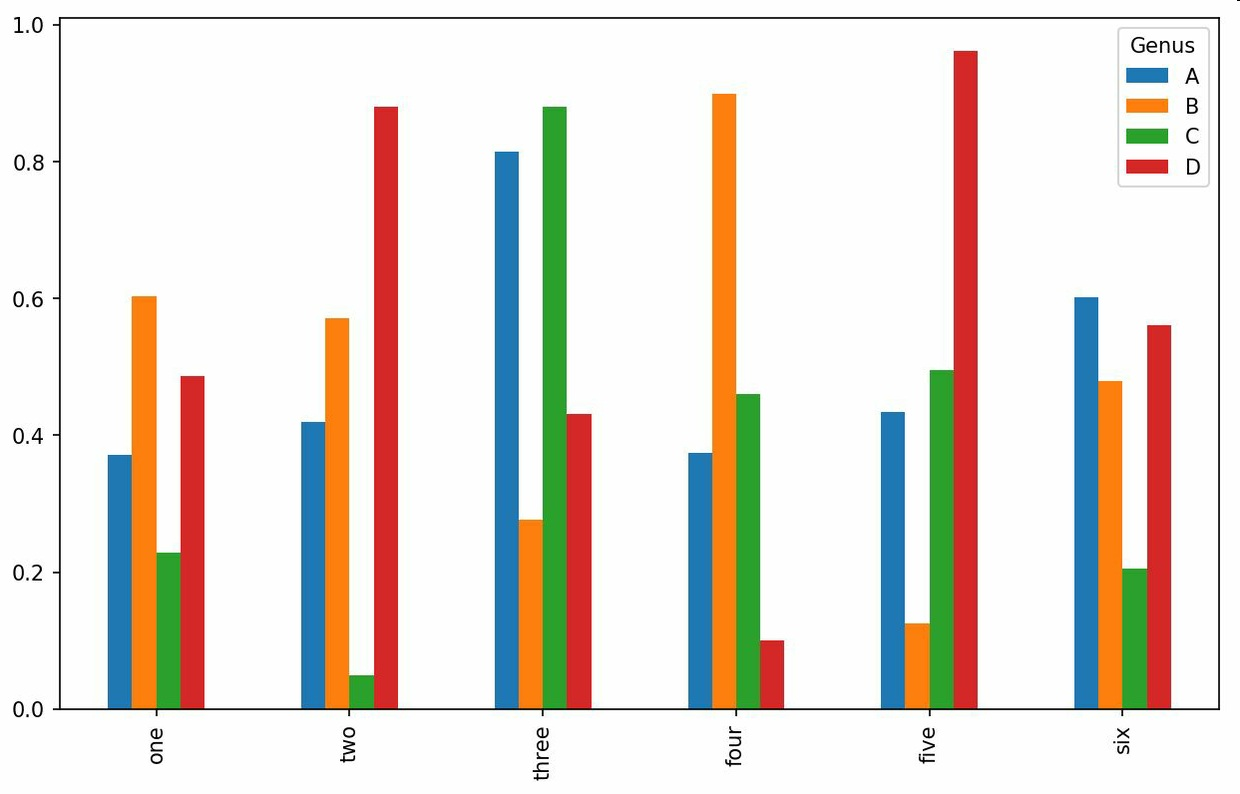### 直方图和密度图

``````In : tips['tip_pct'].plot.hist(bins=50)
``````
• 1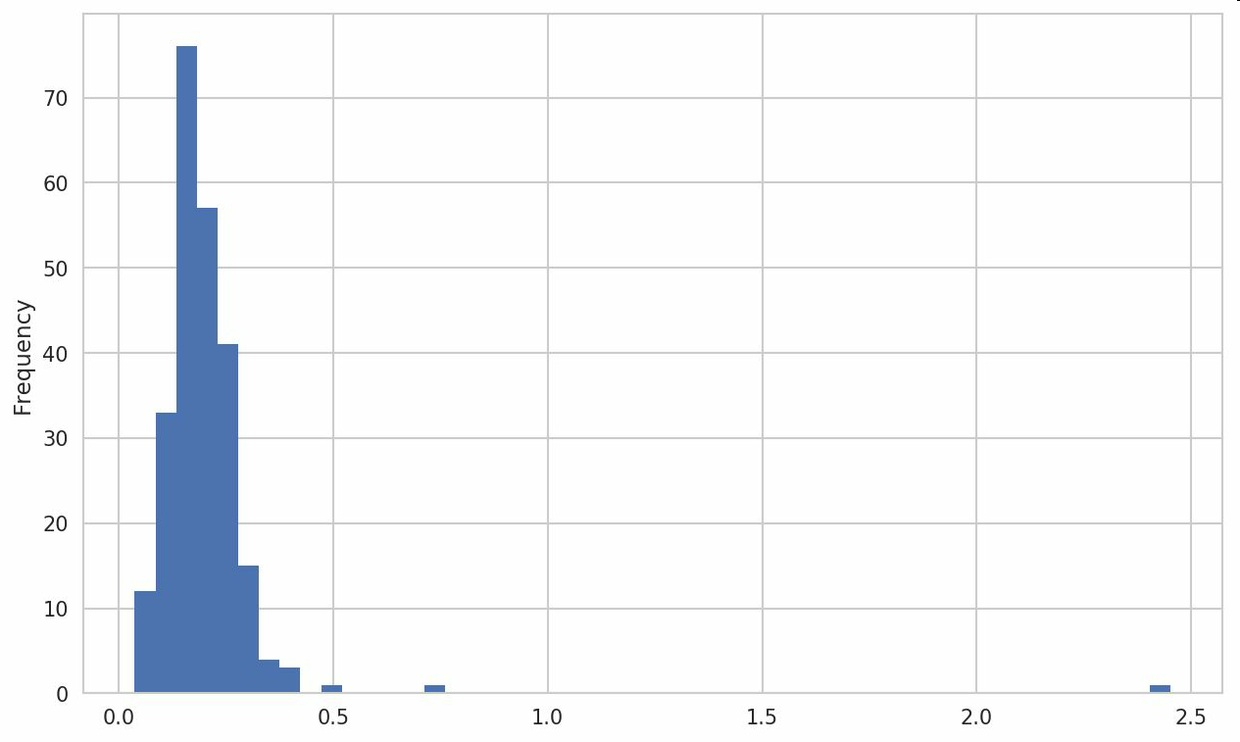``````In : tips['tip_pct'].plot.density()
``````
• 1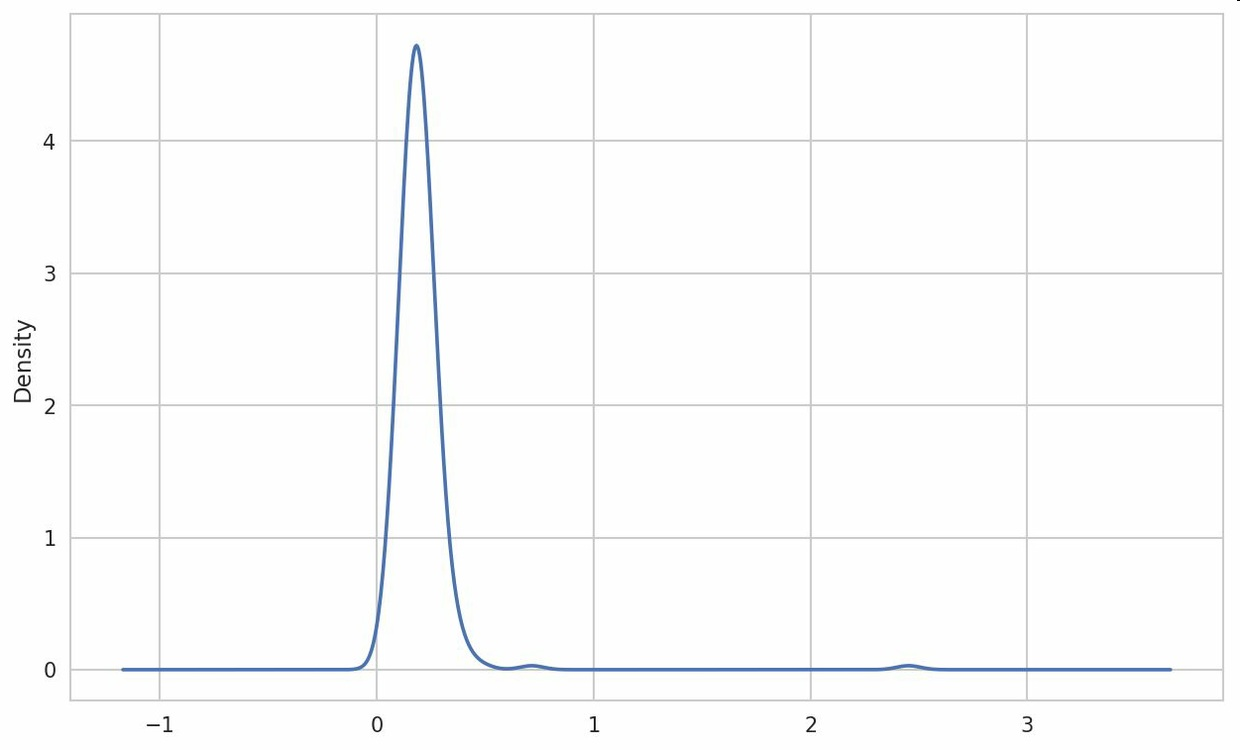可以使用seaborn的regplot方法做一个散布图，在加上一条线性回归：

``````In : sns.regplot('m1', 'unemp', data=trans
_
data)
Out: <matplotlib.axes._
subplots.AxesSubplot at 0x7fb613720b
e0>
In : plt.title('Changes in log %s versus log %s' % ('m1', '
unemp'))
``````
• 1
• 2
• 3
• 4
• 5
• 6
• 7
• 8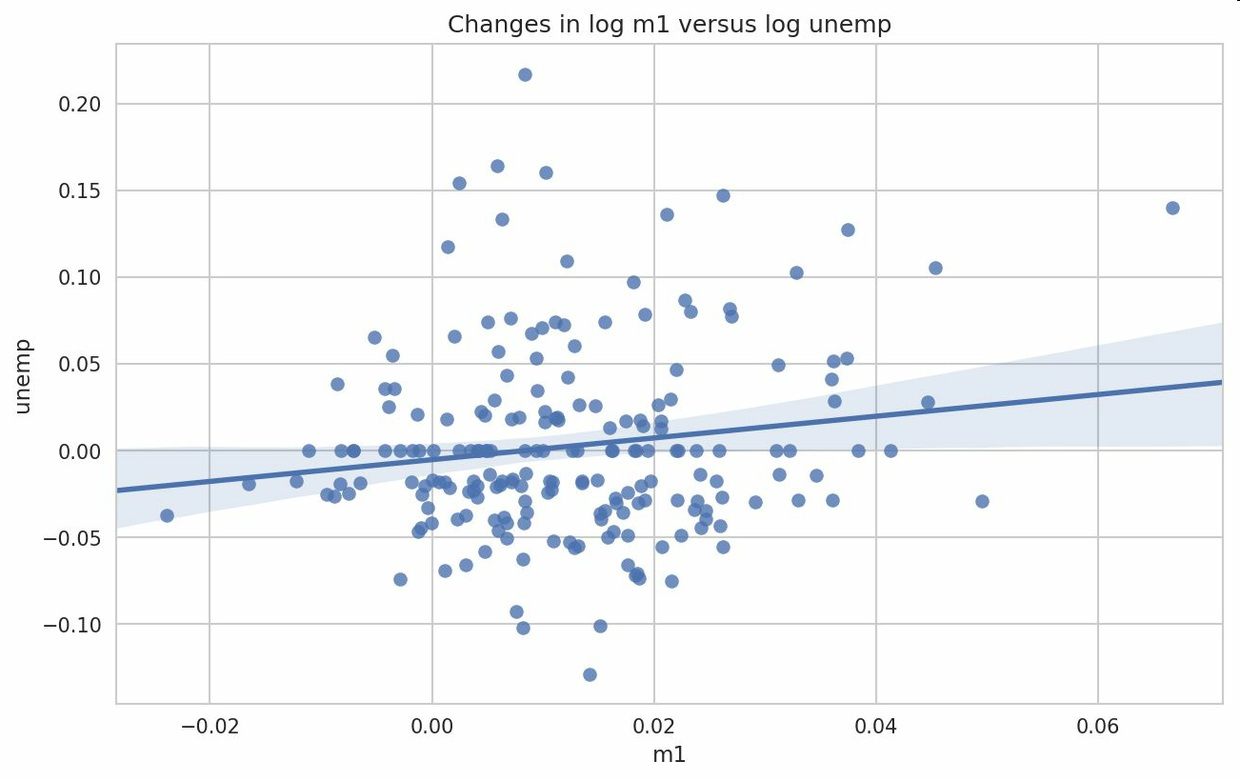### 分面网格和类型数据

``````In : sns.factorplot(x='day', y='tip_pct', hue='time', col=
'smoker',
.....: kind='bar', data=tips[tips.tip_pct < 1]
)
``````
• 1
• 2
• 3
• 4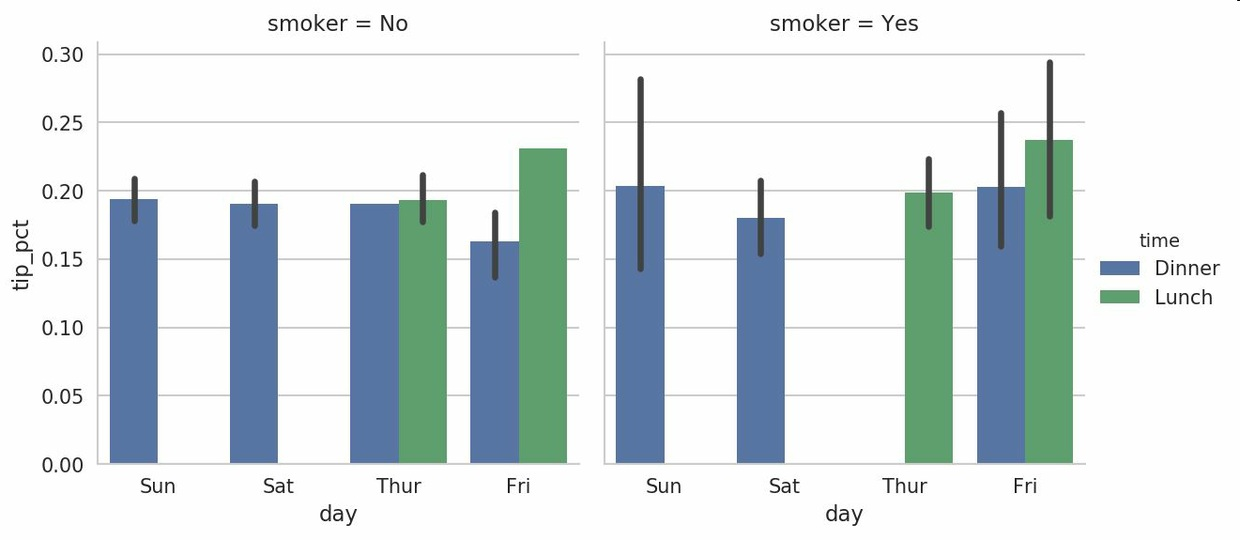``````In : sns.factorplot(x='day', y='tip_pct', row='time',
.....: col='smoker',
.....: kind='bar', data=tips[tips.tip_pct < 1]
)
``````
• 1
• 2
• 3
• 4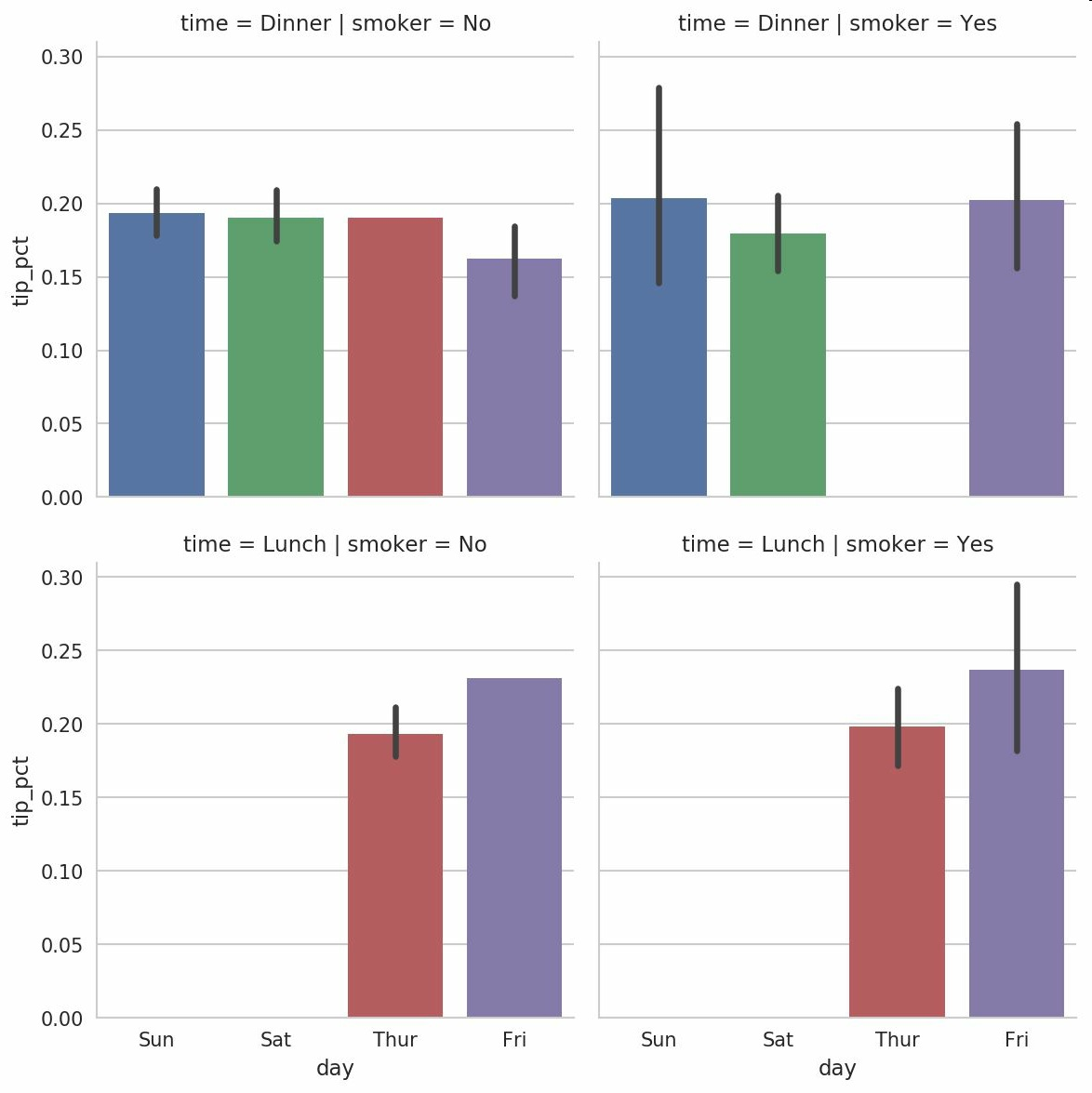factorplot支持其他的绘图类型，盒图

``````In : sns.factorplot(x='tip_pct', y='day', kind='box',
.....: data=tips[tips.tip_pct < 0.5])
``````
• 1
• 2